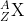×
Get Full Access to Chemistry: A Molecular Approach - 3 Edition - Chapter 2 - Problem 56e
Get Full Access to Chemistry: A Molecular Approach - 3 Edition - Chapter 2 - Problem 56e

×

# Uranium-235 is used in nuclear fission. Determine theISBN: 9780321809247 1

## Solution for problem 56E Chapter 2

Chemistry: A Molecular Approach | 3rd Edition

• Textbook Solutions
• 2901 Step-by-step solutions solved by professors and subject experts
• Get 24/7 help from StudySoup virtual teaching assistantsChemistry: A Molecular Approach | 3rd Edition

4 5 1 299 Reviews
16
3
Problem 56E

Uranium-235 is used in nuclear fission. Determine the number of protons and the number of neutrons in uranium-235 and write its symbol in the form.

Step-by-Step Solution:
Step 1 of 3

Solution : Let’s consider the 1st set of data : The given symbol is P. Phosphorus is the chemical name of symbol P. It has an atomic number of 15. Atomic number indicates the number of protons and electrons. So there are 15 p rotons and electrons. But there is an charge of 3- which indicates there is gain of 3 electrons, Hence number of electrons here is 1 8. Mass number is given as 31. To find the number of neutrons, we subtract the number of protons from the mass number as mass number is the sum of number of protons and neutrons. Therefore, Number of neutrons = 31- 15 = 1 6. Let’s consider the 2nd set of data : Number of protons is given as 34. It indicates the element’s atomic number is 34. Element with atomic number 34 is Selenium(Se). Net charge of the element is given as 2-. This indicates there is gain of 2 electrons as in neutral state number of protons and electrons are equal and hence the element has a charge of 2-. Mass number is the sum of protons and neutrons. Hence mass number = 34+45 = 79. Let’s consider the 3rd set of data : Number of protons is given as 50. It indicates the element’s atomic number is 50. Element with atomic number 50 is T in(Sn). · Number of electrons is given as 46. This indicates there is loss of 4 electrons and hence the net charge of the element is 4+. Mass number is the sum of protons and neutrons. Hence mass number = 69+50 = 119. Let’s consider the 4th set of data : Number of electrons is given as 76 and net charge of the element is given as 3+ , this indicates there is loss of 3 electrons and hence the number of protons is 79. Number of protons gives the atomic number. Hence, element with atomic number 79 is Gold(Au). Mass number is the sum of protons and neutrons. Hence mass number = 79+118 = 197. So finally with all the above derived data, the table is as follows : Symbol 3P 3- 7Se2- 119Sn+ 19Au3+ Protons 15 34 50 79 Neutrons 16 45 69 118 Electrons 18 36 46 76 Net charge 3- 2- 4+ 3+

Step 2 of 3

Step 3 of 3

##### ISBN: 9780321809247

Unlock Textbook Solution